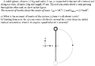# Moment of inertia

• Lord Dark

## Homework Statement

hi again ,, help me to get moment of inertia
A solid sphere, of mass 1.5 kg and radius 5 cm, is connected to the end of a vertical rod
along a y–axis, of mass 2 kg and length 35 cm. The rod can rotate about z–axis passing
through the other end, as show in the figure.

a) What is the moment of inertia of the system (sphere + rod) about z–axis?
b) Starting from rest, the system rotates clockwise around the z–axis from the initial
vertical orientation, what is its angular speed when it is inverted?

## Homework Equations

The moment of inertia about the center of mass:
Irod = ML^2/12 and Isphere = (2/5)mR^2

## The Attempt at a Solution

i tried to solve (I still don't how to get moment of inertia yet) like this :
I=Irod + m(L/2)^2 + (2/5)mR^2+m(LR)^2 = (1/3)ML^2+(7/5)mR^2 is it right ??

#### Attachments

•Q2.JPG
36.5 KB · Views: 360

I=Irod + m(L/2)^2 + (2/5)mR^2+m(LR)^2 = (1/3)ML^2+(7/5)mR^2 is it right ??
Not quite right. You are applying the parallel axis theorem. You did the rod part correctly, but not the sphere. What's the distance from the center of the sphere to the desired axis of rotation?

I=Irod + m(L/2)^2 + (2/5)mR^2+m(L+R)^2 ??

It would help to be able to see the diagram, so maybe you could at least describe it.

In particular: is the sphere connected to the rod at its surface, like the tip of the rod is glued to the surface of the sphere, or is there a hole drilled in the sphere so that the end of the rod coincides with the center of the sphere?

I'm guessing it's the first case, in which case I think you've got it.

I=Irod + m(L/2)^2 + (2/5)mR^2+m(L+R)^2 ??
As belliott4488 explained, that would work. However, lacking any evidence to the contrary, I would choose the simplest arrangement and assume that the center of the sphere is at the end of the rod and thus is a distance L from the axis. Do it both ways. ;-)

In the test they don't care about the simplest , anything they see wrong than their model answer,, I'll get wrong ,, thank guys for checking

In the test they don't care about the simplest , anything they see wrong than their model answer,, I'll get wrong ,, thank guys for checking
In that case the appropriate response is to ask for clarification; the diagram doesn't illustrate the length of the stick L.

Ok ,, I'll ask him ,, if he came anyway :P ,, thanks very much

It would help to be able to see the diagram, so maybe you could at least describe it.
Huh ... I hadn't noticed that diagram when I wrote this response ... did you add it later?

Anyway, if you're putting down a written answer, I would respond with both answers, identifying the assumptions used for each one. As a grader, I would be impressed by your being able to answer either case.

Noted & thanks :)